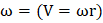# Two identical metal spheres suspended by vertical cords, initially touch each other as shown in fig. Sphereis pulled to the left to a heightand released. After swinging down it collides elastically with sphereThe height undergone by spherewill bea)b) Less thanc) more thand) Zero

## Question ID - 100524 :- Two identical metal spheres suspended by vertical cords, initially touch each other as shown in fig. Sphereis pulled to the left to a heightand released. After swinging down it collides elastically with sphereThe height undergone by spherewill bea)b) Less thanc) more thand) Zero

3537

 (a) The whole of the energy ofwill be transferred toSowill rise to the same height

Next Question :

A uniform solid sphere of radiusis rolling on a smooth horizontal surface with velocityand angular velocity. The sphere collides with a sharp edge on the wall as shown in Figure. The coefficient of friction between the sphere and the edge. Just after the collision the angular velocity of the sphere becomes equal to zero. The linear velocity of the sphere just after the collision is equal toa)b)c)d)